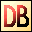DelphiBasicsFracFunction The fractional part of a floating point number System unit
 function Frac ( const Number : Extended ) : Extended;
Description
The Frac function returns the fractional part of a floating point number.
Related commands
 Int The integer part of a floating point number as a float Round Rounds a floating point number to an integer Trunc The integer part of a floating point numberDownload this web site as a Windows program.